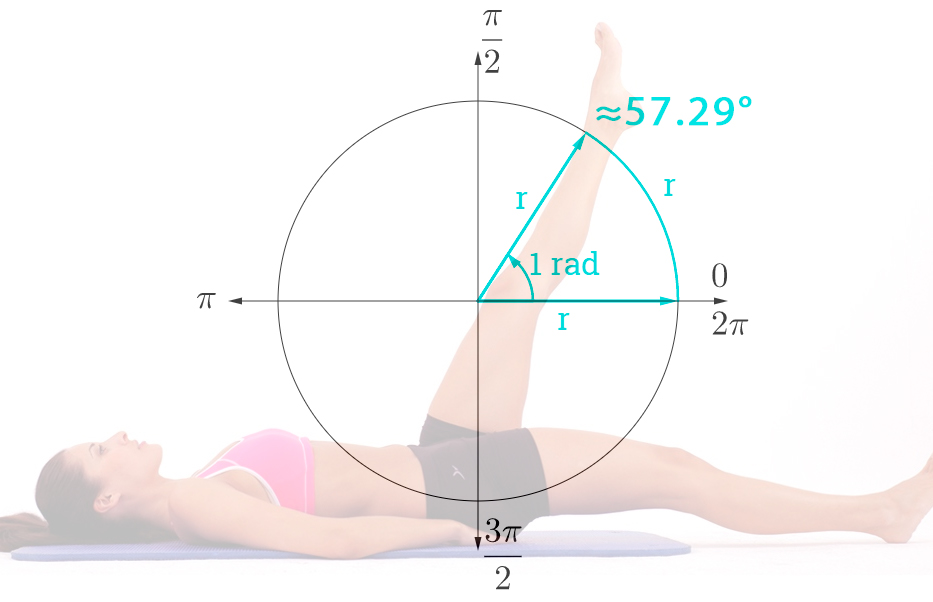The generally accepted and understandable degrees, unfortunately, are a non-systematic unit of measure for angle. In the scientific environment, radians are used to measure angles. One degree (1°) is roughly equal to 0.0174533 radians (rad). It is quite difficult to convert degrees to radians in your head, which is why we created this simple, fast and convenient online calculator. Two clicks and you get the required numerical value.This is what a radian looks like on a circle

## Pre-performed calculations

• Degrees = °
 ° rad rad (via π) 180 3.1415926535 π 360 6.2831853070 2π 90 1.5707963268 π/2 15 0.2617993878 π/12 30 0.5235987756 π/6 45 0.7853981634 π/4 60 1.0471975512 π/3 75 1.3089969390 π/2.4 120 2.0943951023 2π/3 135 2.3561944901 3π/4 150 2.6179938779 5π/6 270 4.7123889803 3π/2

### Formula to convert ° to rad

To convert degrees to radians, you need to multiply the number of degrees by π and divide by 180:

### Formula to convert rad to °

To convert radians to degrees, you need to multiply the number of radians by 180 and divide by π: